# Neural networks for algorithmic trading. Correct time series forecasting + backtesting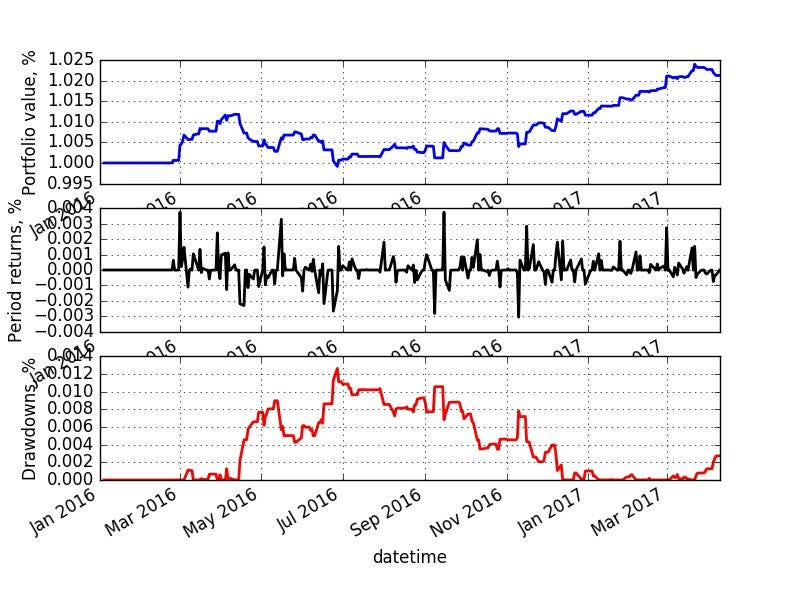Not that good, right?

Hi everyone! Some time ago I published a small tutorial on financial time series forecasting which was interesting, but in some moments wrong. I have spent some time working with different time series of different nature (applying NNs mostly) in HPA, that particularly focuses on financial analytics, and in this post I want to describe more correct way of working with financial data. Comparing to previous post, I want to show different way of data normalizing and discuss more issues of overfitting (which definitely appears while working with data that has stochastic nature). We won’t compare different architectures (CNN, LSTM), you can check them in previous post. But even working only with simple feed-forward neural nets we will see important things. If you want to jump directly to the code — check out IPython Notebook. For Russian speaking readers, it’s a translation of my post here and you can check webinar on backtesting here.

Other posts are here:

### Data preparation

Let’s take historical time series of Apple stock prices starting from 2005 till today. You can easily download them from Yahoo Finance as .csv file. In this file data is in “reversed” order — from 2017 till 2005, so we need to reverse it back first and have a look:

`data = pd.read_csv('./data/AAPL.csv')[::-1] close_price = data.ix[:, 'Adj Close'].tolist() plt.plot(close_price) plt.show()`

As we discussed in previous post, we can treat problem of financial time series forecasting in two different ways (let’s omit volatility forecasting, anomaly detection and other interesting things for now):

We will consider our problem as 1) regression problem (trying to forecast exactly close price or return next day) 2) binary classification problem (price will go up [1; 0] or down [0; 1]).
First let’s prepare our data for training. We want to predict t+1 value based on N previous days information. For example, having close prices from past 30 days on the market we want to predict, what price will be tomorrow, on the 31st day. We use first 90% of time series as training set (consider it as historical data) and last 10% as testing set for model evaluation.

The main problem of financial time series — they’re not stationary, which means, that their statistical properties (mean, variance, maximal and minimal values) change over time and we can check it with augmented Dickey-Fuller test. And because of this we can’t use classical data normalization methods like MinMax or Z-score normalization.

In our case, we will cheat a bit for classification problem. We don’t need to predict some exact value, so expected value and variance of the future isn’t very interesting for us — we just need to predict the movement up or down. That’s why we will risk and normalize our 30-days windows only by their mean and variance (z-score normalization), supposing that just during single time window they don’t change much and not touching information from the future:

`X = [(np.array(x) — np.mean(x)) / np.std(x) for x in X]`

For regression problem we already can’t cheat like this, so will use returns (percentage of how much price changed comparing to yesterday) with pandas and it looks like:

`close_price_diffs = close.price.pct_change()`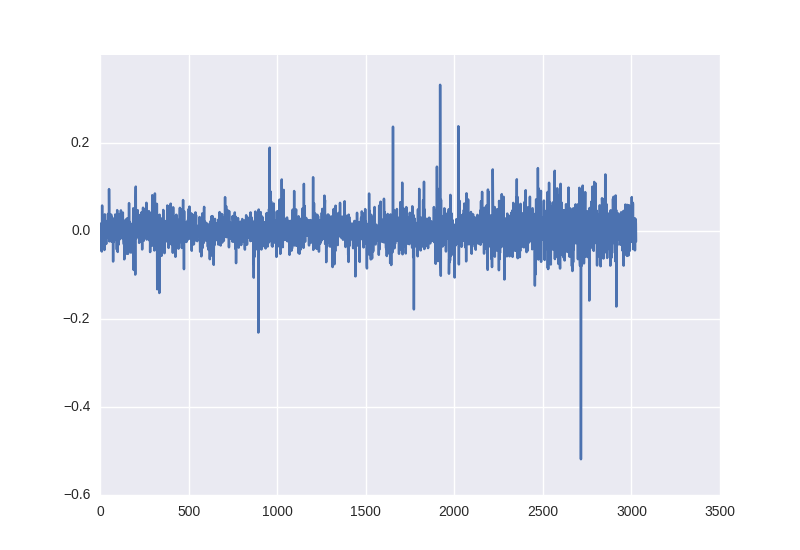Daily returns of Apple stock over time

As we can see, this data is already normalized and lies from -0.5 to 0.5.

### Neural network architecture

As I said before, we will work only with MLPs in this article to show how easy to overfit neural networks on financial data (and actually what happened in previous post) and how to prevent it. Expand these ideas on CNNs or RNNs will be relatively easy, but it’s much more important to understand the concept. As before, we use Keras as main framework for neural nets prototyping.

Our first net will look like this:

`model = Sequential() model.add(Dense(64, input_dim=30)) model.add(BatchNormalization()) model.add(LeakyReLU()) model.add(Dense(2)) model.add(Activation('softmax'))`

I can suggest always use Batch normalization after every affine or convolutional layer and Leaky ReLU as basic activation function, just because it’s already became “industrial standard” — they help to train nets way much faster. Other nice thing is reducing learning rate during training, Keras makes this with ReduceLROnPlateau:

`reduce_lr = ReduceLROnPlateau(monitor='val_loss', factor=0.9, patience=5, min_lr=0.000001, verbose=1) `
`model.compile(optimizer=opt,                loss='categorical_crossentropy', metrics=['accuracy'])`

This is how we launch training:

`history = model.fit(X_train, Y_train, nb_epoch = 50,            batch_size = 128, verbose=1, validation_data=(X_test, Y_test),           shuffle=True, callbacks=[reduce_lr])`

And this is how we will visualize results (let’s judge loss and accuracy plots)

`plt.figure() plt.plot(history.history['loss']) plt.plot(history.history['val_loss']) plt.title('model loss') plt.ylabel('loss') plt.xlabel('epoch') plt.legend(['train', 'test'], loc='best') `
Important moment: in last post we trained our nets only for 10 epochs — it’s completely wrong. Even we can see 55% of accuracy, it doesn’t really mean that we can predict future better than random. Most probably, in our dataset we just have 55% of time windows with one behavior (up) and 45% with another (down). And our network only learn this distribution of training data. So it’s better to learn them fro 20–50–100 epochs and if it’s too much to use early stopping.

### Classification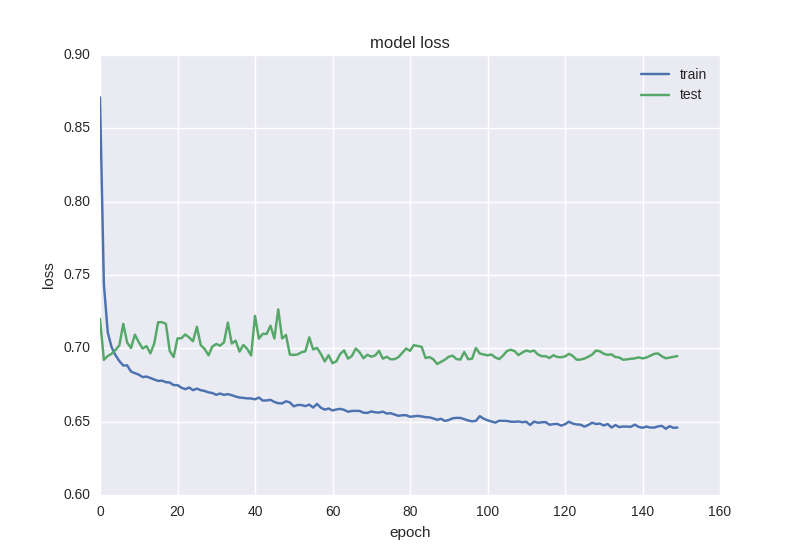First network loss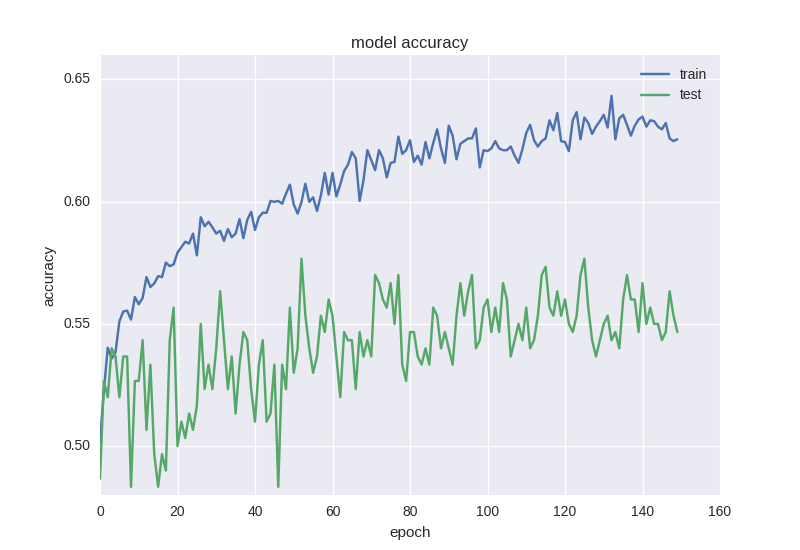First network accuracy

The results aren’t good at all, our test loss doesn’t change at all, we can see clear overfit, let’s make a deeper network and try it:

`model = Sequential() model.add(Dense(64, input_dim=30)) model.add(BatchNormalization()) model.add(LeakyReLU()) model.add(Dense(16)) model.add(BatchNormalization()) model.add(LeakyReLU()) model.add(Dense(2)) model.add(Activation('softmax'))`

Here are results: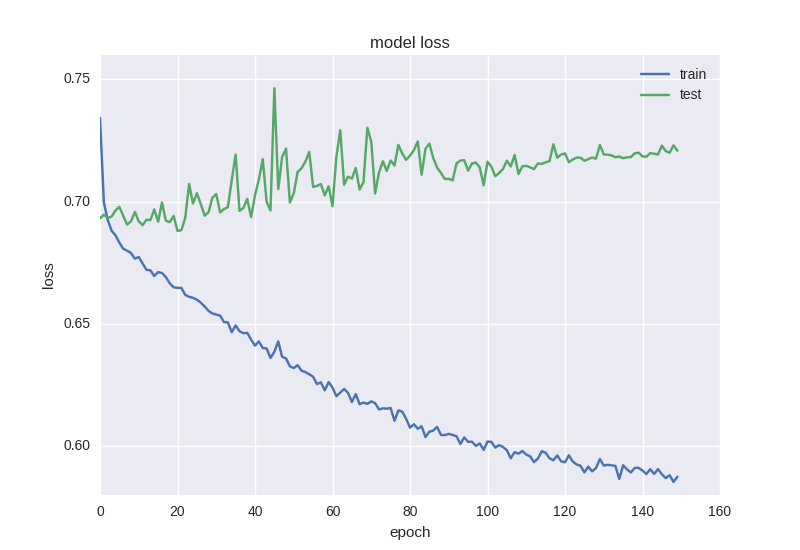Second network loss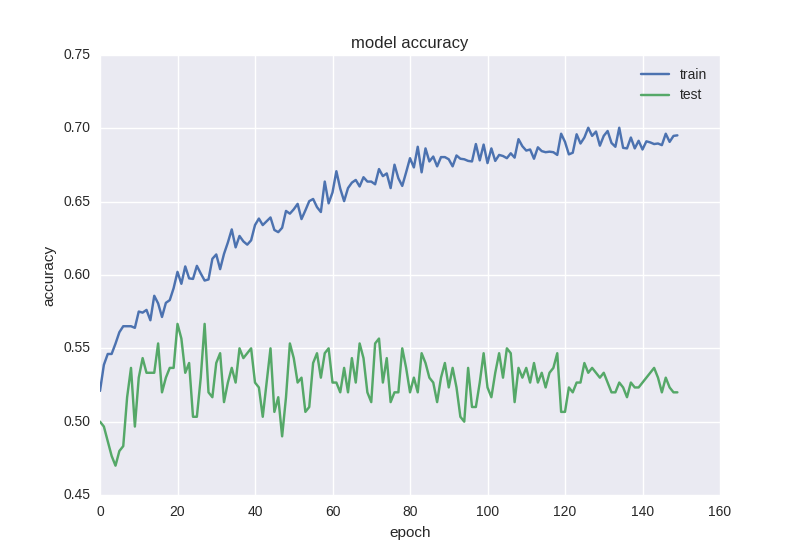Second network accuracy

Here we see more or less the same, even worse… It’s time to add some regularization to the model, starting with adding L2 norm on sum of weights:

`model = Sequential() model.add(Dense(64, input_dim=30,                 activity_regularizer=regularizers.l2(0.01))) model.add(BatchNormalization()) model.add(LeakyReLU()) model.add(Dense(16,                 activity_regularizer=regularizers.l2(0.01))) model.add(BatchNormalization()) model.add(LeakyReLU()) model.add(Dense(2)) model.add(Activation('softmax'))`

It works better, but still not good enough (even loss is decreasing, but accuracy is bad). It’s happening very often while working with financial data — it’s explained very nicely here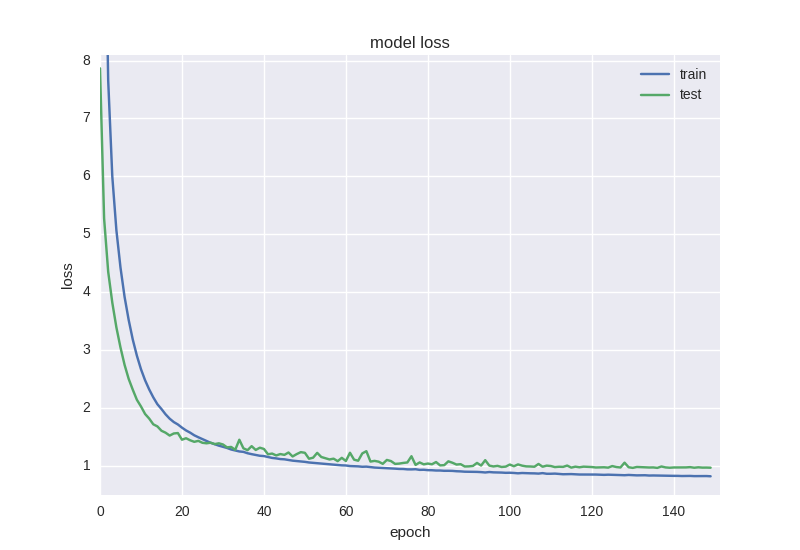Regularized network loss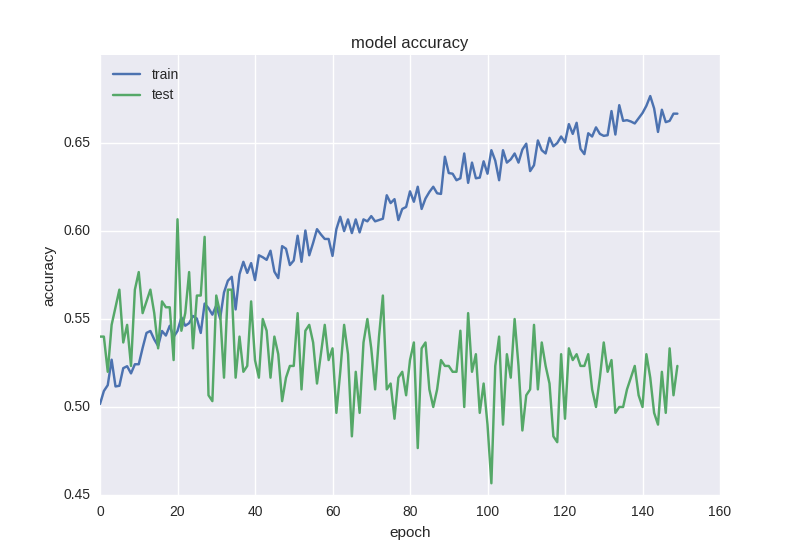Regularized network accuracy

The next thing I want to do looks very weird, but we gonna regularize already regularized network adding hardcore dropout with 0.5 rate (it’s random ignoring some weights while backpropagation to avoid neurons coadaptation and therefore overfitting):

`model = Sequential()  model.add(Dense(64, input_dim=30,                 activity_regularizer=regularizers.l2(0.01))) model.add(BatchNormalization()) model.add(LeakyReLU()) model.add(Dropout(0.5)) model.add(Dense(16,                 activity_regularizer=regularizers.l2(0.01))) model.add(BatchNormalization()) model.add(LeakyReLU()) model.add(Dense(2)) model.add(Activation('softmax'))`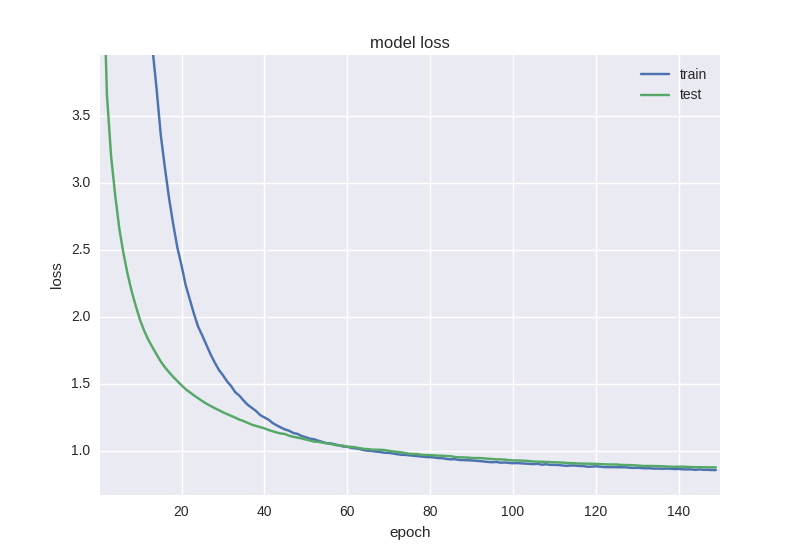Hardcore regularized network loss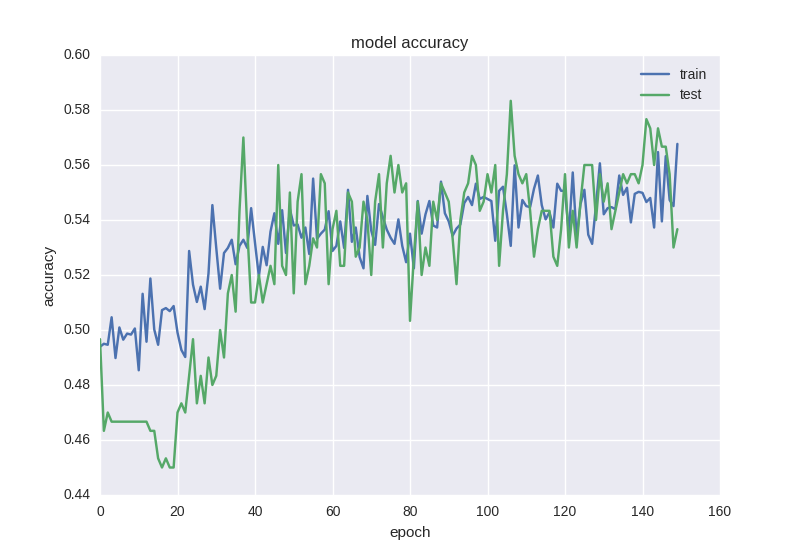Hardcore regularized network accuracy

As we can see, plots look more or less adequate and we can report about 58% of accuracy, which is slightly better than random guessing.

Try just for fun to learn network to forecast movement not the next day, but in five days (is the price higher or lower in 5 days comparing to today). Does it work better? If it works better — why?

### Regression

For regression, we will use returns data, previous successful neural network architecture (but without dropouts) and check how regression works:

`model = Sequential()model.add(Dense(64, input_dim=30,                 activity_regularizer=regularizers.l2(0.01))) model.add(BatchNormalization()) model.add(LeakyReLU()) model.add(Dense(16,                 activity_regularizer=regularizers.l2(0.01))) model.add(BatchNormalization()) model.add(LeakyReLU()) model.add(Dense(1)) model.add(Activation('linear'))`

And here is code for plotting forecasts visually:

`pred = model.predict(np.array(X_test)) original = Y_test predicted = pred  `
`plt.plot(original, color='black', label = 'Original data') plt.plot(predicted, color='blue', label = 'Predicted data') plt.legend(loc='best') plt.title('Actual and predicted') plt.show()`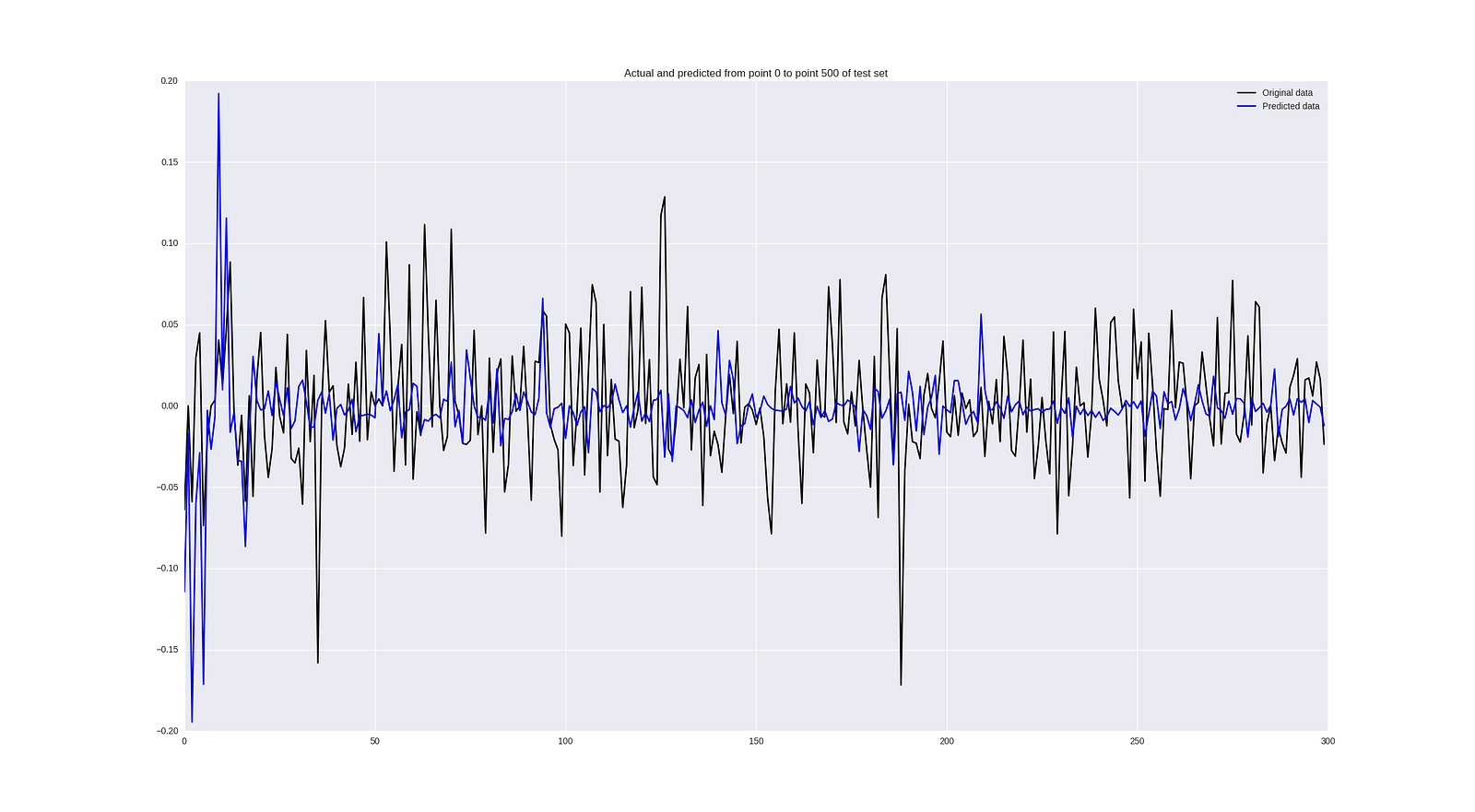Returns forecast

It works simply bad, even isn’t worth to comment it. I will tell some tips that can help with regression problem in conclusion part.

### Backtesting

Let’s remember why are we messing with all these time series in general? We want to build a trading system, which means, it has to make some deals — buy, sell stocks and, hopefully, grow your portfolio.

There are a lot of good ready solutions to backtest your strategies (like Quantopian), but I decided to learn how they’re built from inside and bought the following book with details of implementation (not a product placement ahahah):

The strategy I’ve tested is extremely simple: if our network says that price will go up, we buy the stock and sell it only after network says that price will go down and will wait for the next buying signal. The logic looks like:

`if np.argmax(pred) == 0 and not self.long_market:     self.long_market = True     signal = SignalEvent(1, sym, dt, 'LONG', 1.0)     self.events.put(signal)     print pred, 'LONG'if np.argmax(pred) == 1 and self.long_market:     self.long_market = False     signal = SignalEvent(1, sym, dt, 'EXIT', 1.0)     self.events.put(signal)     print pred, 'EXIT'`

Here are the results of training classification network on data from 2012 to 2016 and testing from 2016 to the May of 2017:Trading results

Blue plot shows portfolio value growth (wow, 3% in 1.5 years), black shows “activity” and red one — drawdowns (periods of losing money).

### Discussion

On the first glimpse, results are bad. Horrible regression and not really amazing classification (58% of accuracy) are asking us to leave this idea. And after seeing that “incredible” 3% income (it would be easier just to buy Apple stocks and hold, they grew in 20% for that time) you maybe want to close laptop and do something that doesn’t involve finance or machine learning. But there are lot of ways to improve our results (and what people do in funds):

• Use high frequency data (hourly, minute ticks) — machine learning algorithms need more data and predict better on short distance
• Do smart hyperparameter optimization including not only neural network optimization and training parameters, but also historical time window(s) you train on
• Use better architectures of neural networks like CNNs or RNNs
• Use not only closing price or returns, but all OHLCV tuple for every day; if it’s possible — collect information about N most correlated compamies, sector financial status, economical variables etc. It’s impossible to build good forecasting model relying on that simple data we used
• Use more sophisticated, maybe assymetric, loss functions. For example MSE that we used for regression is invariant to the sign, which is crucial for our task.

### Conclusion

Forecasting of financial data is extremely complicated. It’s easy to overfit, we don’t know correct historical range to train on and it’s difficult to get all data needed. But as we can see, it works, and even can give some profits. This article can be good starting point and pipeline for further research and discovery.

In next posts I plan to show automated hyperparameter search process, add more data (full OHLCV and financial indicators), apply reinforcement learning to learn the strategy and check if reinforcement agent will trust our predictions. Stay tuned!

P.S.
Follow me also in Facebook for AI articles that are too short for Medium, Instagram for personal stuff and Linkedin!